1669952228

# Dijkstra's Algorithm Explained with Examples

### In this tutorial, you'll learn: What is Dijkstra's Algorithm and how Dijkstra's algorithm works with the help of visual guides.

You can use algorithms in programming to solve specific problems through a set of precise instructions or procedures.

Dijkstra's algorithm is one of many graph algorithms you'll come across. It is used to find the shortest path from a fixed node to all other nodes in a graph.

There are different representations of Dijkstra's algorithm. You can either find the shortest path between two nodes, or the shortest path from a fixed node to the rest of the nodes in a graph.

In this article, you'll learn how Dijkstra's algorithm works with the help of visual guides.

## How Does Dijkstra’s Algorithm Work?

Before we dive into more detailed visual examples, you need to understand how Dijkstra's algorithm works.

Although the theoretical explanation may seem a bit abstract, it'll help you understand the practical aspect better.

In a given graph containing different nodes, we are required to get the shortest path from a given node to the rest of the nodes.

These nodes can represent any object like the names of cities, letters, and so on.

Between each node is a number denoting the distance between two nodes, as you can see in the image below:We usually work with two arrays – one for visited nodes, and another for unvisited nodes. You'll learn more about the arrays in the next section.

When a node is visited, the algorithm calculates how long it took to get to the node and stores the distance. If a shorter path to a node is found, the initial value assigned for the distance is updated.

Note that a node cannot be visited twice.

The algorithm runs recursively until all the nodes have been visited.

## Dijkstra's Algorithm Example

In this section, we'll take a look at a practical example that shows how Dijkstra's algorithm works.

Here's the graph we'll be working with:We'll use the table below to put down the visited nodes and their distance from the fixed node:

Visited nodes = []
Unvisited nodes = [A,B,C,D,E]

Above, we have a table showing each node and the shortest distance from the that node to the fixed node. We are yet to choose the fixed node.

Note that the distance for each node in the table is currently denoted as infinity (∞). This is because we don't know the shortest distance yet.

We also have two arrays – visited and unvisited. Whenever a node is visited, it is added to the visited nodes array.

Let's get started!

To simplify things, I'll break the process down into iterations. You'll see what happens in each step with the aid of diagrams.

### Iteration #1

The first iteration might seem confusing, but that's totally fine. Once we start repeating the process in each iteration, you'll have a clearer picture of how the algorithm works.

Step #1 - Pick an unvisited node

We'll choose A as the fixed node. So we'll find the shortest distance from A to every other node in the graph.We're going to give A a distance of 0 because it is the initial node. So the table would look like this:

Step #2 - Find the distance from current nodeThe next thing to do after choosing a node is to find the distance from it to the unvisited nodes around it.

The two unvisited nodes directly linked to A are B and C.

To get the distance from A to B:

0 + 4 = 4

0 being the value of the current node (A), and 4 being the distance between A and B in the graph.

To get the distance from A to C:

0 + 2 = 2

Step #3 - Update table with known distances

In the last step, we got 4 and 2 as the values of B and C respectively. So we'll update the table with those values:

Step #4 - Update arrays

At this point, the first iteration is complete. We'll move node A to the visited nodes array:

Visited nodes = [A]
Unvisited nodes = [B,C,D,E]

Before we proceed to the next iteration, you should know the following:

• Once a node has been visited, it cannot be linked to the current node. Refer to step #2 in the iteration above and step #2 in the next iteration.
• A node cannot be visited twice.
• You can only update the shortest known distance if you get a value smaller than the recorded distance.

### Iteration #2

Step #1 - Pick an unvisited node

We have four unvisited nodes — [B,C,D,E]. So how do you know which node to pick for the next iteration?

Well, we pick the node with the smallest known distance recorded in the table. Here's the table:

So we're going with node C.Step #2 - Find the distance from current node

To find the distance from the current node to the fixed node, we have to consider the nodes linked to the current node.

The nodes linked to the current node are A and B.

But A has been visited in the previous iteration so it will not be linked to the current node. That is:From the diagram above,

• The green color denotes the current node.
• The blue color denotes the visited nodes. We cannot link to them or visit them again.
• The red color shows the link from the unvisited nodes to the current node.

To find the distance from C to B:

2 + 1 = 3

2 above is recorded distance for node C while 1 is the distance between C and B in the graph.

Step #3 - Update table with known distances

In the last step, we got the value of B to be 3. In the first iteration, it was 4.

We're going to update the distance in the table to 3.

So, A --> B = 4 (First iteration).

A --> C --> B = 3 (Second iteration).

The algorithm has helped us find the shortest path to B from A.

Step #4 - Update arrays

We're done with the last visited node. Let's add it to the visited nodes array:

Visited nodes = [A,C]
Unvisited nodes = [B,D,E]

### Iteration #3

Step #1 - Pick an unvisited node

We're down to three unvisited nodes — [B,D,E]. From the array, B has the shortest known distance.To restate what is going on in the diagram above:

• The green color denotes the current node.
• The blue color denotes the visited nodes. We cannot link to them or visit them again.
• The red color shows the link from the unvisited nodes to the current node.

Step #2 - Find the distance from current node

The nodes linked to the current node are D and E.

B (the current node) has a value of 3. Therefore,

For node D, 3 + 3 = 6.

For node E, 3 + 2 = 5.

Step #3 - Update table with known distances

Step #4 - Update arrays

Visited nodes = [A,C,B]
Unvisited nodes = [D,E]

### Iteration #4

Step #1 - Pick an unvisited node

Like other iterations, we'll go with the unvisited node with the shortest known distance. That is E.Step #2 - Find the distance from current node

According to our table, E has a value of 5.

For D in the current iteration,

5 + 5 = 10.

The value gotten for D here is 10, which is greater than the recorded value of 6 in the previous iteration. For this reason, we'll not update the table.

Step #3 - Update table with known distances

Our table remains the same:

Step #4 - Update arrays

Visited nodes = [A,C,B,E]
Unvisited nodes = [D]

### Iteration #5

Step #1 - Pick an unvisited node

We're currently left with one node in the unvisited array — D.Step #2 - Find the distance from current node

The algorithm has gotten to the last iteration. This is because all nodes linked to the current node have been visited already so we can't link to them.

Step #3 - Update table with known distances

Our table remains the same:

At this point, we have updated the table with the shortest distance from the fixed node to every other node in the graph.

Step #4 - Update arrays

Visited nodes = [A,C,B,E,D]
Unvisited nodes = []

As can be seen above, we have no nodes left to visit. Using Dijkstra's algorithm, we've found the shortest distance from the fixed node to others nodes in the graph.

## Dijkstra's Algorithm Pseudocode Example

The pseudocode example in this section was gotten from Wikipedia. Here it is:

`````` 1  function Dijkstra(Graph, source):
2
3      for each vertex v in Graph.Vertices:
4          dist[v] ← INFINITY
5          prev[v] ← UNDEFINED
7      dist[source] ← 0
8
9      while Q is not empty:
10          u ← vertex in Q with min dist[u]
11          remove u from Q
12
13          for each neighbor v of u still in Q:
14              alt ← dist[u] + Graph.Edges(u, v)
15              if alt < dist[v]:
16                  dist[v] ← alt
17                  prev[v] ← u
18
19      return dist[], prev[]``````

## Applications of Dijkstra's Algorithm

Here are some of the common applications of Dijkstra's algorithm:

• In maps to get the shortest distance between locations. An example is Google Maps.
• In telecommunications to determine transmission rate.
• In robotic design to determine shortest path for automated robots.

## Summary

In this article, we talked about Dijkstra's algorithm. It is used to find the shortest distance from a fixed node to all other nodes in a graph.

We started by giving a brief summary of how the algorithm works.

We then had a look at an example that further explained Dijkstra's algorithm in steps using visual guides.

We concluded with a pseudocode example and some of the applications of Dijkstra's algorithm.

Happy coding!

Original article source at https://www.freecodecamp.org

#algorithm #datastructures

## Buddha Community1624867080

## Algorithm trading backtest and optimization examplesAlgorithm trading backtest and optimization examples.

### xbtusd-vanila-market-making-backtest-hedge1593762420

## Pathfinding: Dijkstra’s Algorithm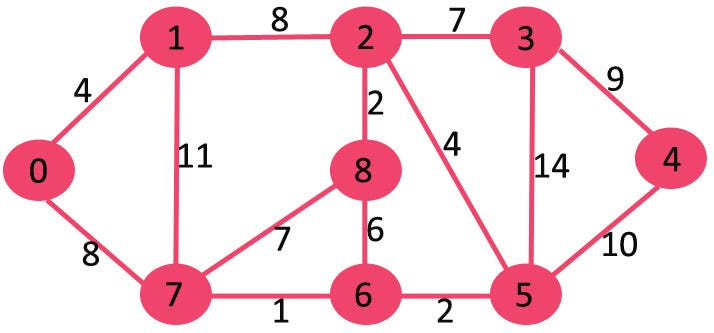I like tower defense games and was thinking about trying my hand at making one. But while I was making a mental checklist of things I’d need for it, it occurred to me that I would need to study pathfinding algorithms. I didn’t know the best place to start, so I went with a Google search and found the A* algorithm. Neat algorithm, but I did find plenty of notes saying that it was sort of a descendant of Dijkstra’s algorithm, and I found that was a better starting point.

You might want to know a bit about data structures, nodes, and graphs before going into this, but even if you do not, I’ll do my best to bring in as many visuals as I can and keep each step as clear as possible. I’ll also list all the references I found, and credit them as much as I can, because I am totally going to be stealing their examples.

# Set-up

I’ve looked at lot of of graphs today, but I think this is the best and simplest for demonstrating Dijkstra’s algorithm. Just five nodes, or vertices because this is a graph, each with two to four edges.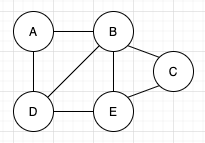“Karl, you even labeled the vertices the same as your source! That’s plagiarism!” Nonsense, I labeled them A through E, left to right, top to bottom. And so did they! Great minds think a like!

Right now, I could ask you what the quickest way from A to C would be is, and you might see that the best path is A to B to C. But graphs are commonly abstractions of some relationship, and in the case of pathfinding, the vertices are specific locations and the edges are the distances between them. So what if we represented longer distances between vertices with longer edges, something like this: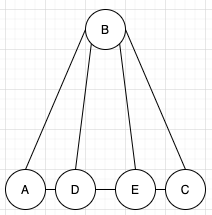As far as graph theory and topology are concerned, this graph is identical to the first one. All the vertices still point to the same vertices as before, but some of the edges are longer and some are shorter. Also, the shortest distance between A and C now passes through D and E.

However, having a graph that is more visually accurate of what it’s representing is not particularly useful in computing anything. Unsurprisingly, the best way to make this graph more algorithmically friendly is to add numbers to it, in the form of weights for the edges.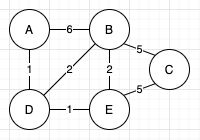The numbers on the edges are called weights, and they’ll represent some value about the relationship between the two vertices. In pathfinding, this will likely be distance. Again, because of the smaller weights, the shortest distance between A and C is still A > D > E > C.

This will be the graph, vertices, and weights I’ll be using for the rest of this article. The goal will be using Dijkstra’s algorithm to find the shortest path between vertices A and C. To do this, I’ll need just a few extra things; some for helping the algorithm, and some to act as visual aides. On the algorithm side, I need two sets to hold the vertices, one called “visited” and one called “unvisited”. The algorithm is going to work through the unvisited vertex set, one at a time, and when it’s done with each vertex, it will place them in the visited set. On the visual aide side, I’ll want a table of all the vertices, to keep track of their shortest distance from vertex A, and the first vertex that was in-between them and vertex A. The final set-up will look something like this: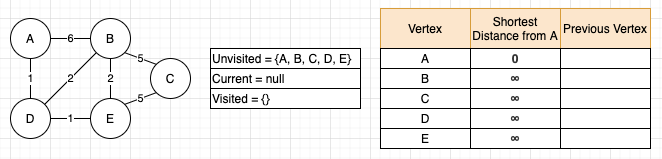A few more things to note here: I’ve jumped ahead a bit and filled in the initial shortest distances. Vertex A starts at zero because if your starting point and ending point are the same, there is no distance to travel. For the rest of the vertices, the distances are initially unknown, and until those are visited and evaluated, they’ll start off as infinity. Second thing to note is that I added a “current” field. This is a more of a visual aide thing to let you know which vertex is currently being visited. And third, this set-up is wholesale theft. I have no excuse for this one, but I’ll leave a link to my source in the references.

# Stepping Through the Algorithm

The first step of the algorithm is to go through the unvisited vertices and look for the one with the shortest distance from A. At the start, everything is unvisited, and the only vertex with a known distance is A, so we start by examining A.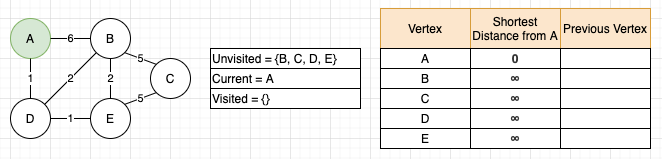Now that we have a current vertex, we need to look at its unvisited neighbors, which in this case are vertices B and D. For each unvisited neighbor, we need to find their distance from vertex A. This will be more complicated later, but because we are currently measuring directly from A to these vertices, it will just be the weights on those edges: A to B is 6, and A to D is 1. With these two values in hand, we need to compare them to the current recorded shortest distance from vertex A, and if the new distance is smaller, replace that value. On the table, we can see that both B and D are still set to infinity, so 6 and 1 are definitely smaller and will be made the new shortest distance. Alongside updating the distance, we also need to record that this new shortest distance was made by a connection through the current vertex. Again, this feels silly on the first loop when it’s a clear, direct connection to A, but this is what makes the algorithm work. Finally, we can move A to the visited set, and that is one loop of the algorithm.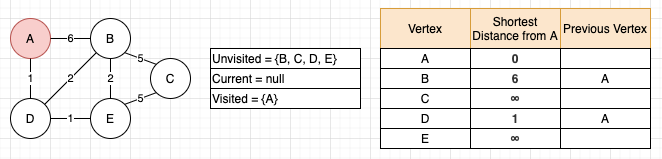# Rinse and Repeat

And now we start all over, going through the same steps and following the same rules. So, look at the unvisited set and find the one with the shortest distance from A. More choices this time around, but D, with a distance of 1, is clearly the shortest, so it will be the new current vertex.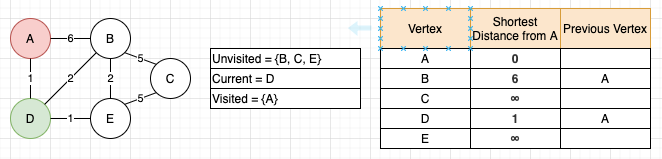Just like before, we now need to look at D’s unvisited neighbors, which are B and E. A is also a neighbor, but because it has been visited already and is in the visited set, it will not be examined, affected, or changed here. Next, we need the distance from the neighbors to A. Using E as an example, the distance from D to E on the graph is 1, and D’s shortest distance from A on the table is 1, so 1+1=2. Before, in the first loop with B and D, this would’ve looked like 0+6 and 0+1, because A was the current vertex and its shortest distance was 0. However, writing out 0+1 in that first pass just looks odd to me, so I passed over explaining it like this until now as I think it makes a little more sense on every iteration after the first.

#dijkstra #pathfinding #algorithms #graph #algorithms1593392340

## Dijkstra’s shortest path algorithm

In 1956 Edsger Wybe Dijkstra introduced an algorithm that aims to find a shortest path in a graph from one node to another, without revisiting any nodes. This algorithm is taught to aspiring computer scientists all over the world, and I find it very interesting.

So here is how it works. Given a graph (for example) with nodes ‘A’, ‘B’, ‘C’, ‘D’, ‘E’, can we find the shortest path from ‘A’ to ‘E’ by taking one step at a time?

#algorithms #computer-science #dijkstra #mathematics #algorithms1626459600

## Introduction

Genetic Algorithm (GA) can sometimes be a bit difficult to understand !! :(

In this article, I’ll help you understand GA with a simple example. So don’t worry. Hang tight. All will be clear soon !! :)

Below are the steps to be followed to solve any optimization problem with the help of GA.

• Step 1- Choose an encoding technique, a selection operator, and a crossover operator
• Step 2- Choose a population size
• Step 3- Randomly choose the initial population
• Step 4- Select parental chromosomes
• Step 5- Perform Crossover (random crossover points)
• Step 6- Evaluation of offsprings
• Step 7- Repeat the process

Now we’ll see an example of a simple optimization problem and try to solve it with the help of the steps mentioned above.

#evolutionary-algorithms #data-science #genetic-algorithm #example1595551620

## Minimum Cost using Dijkstra by reducing cost of an Edge

Given an undirected graph of N nodes and M edges of the form {X, Y, Z} such that there is an edge between X and Y with cost Z. The task is to find the minimum cost to traverse from source node 1 to destination node N such we are allowed to reduce the cost of only one path during traversal by 2.

Examples:

Input:_ N = 3, M = 4, Edges = {{1, 2, 3}, {2, 3, 1}, {1, 3, 7}, {2, 1, 5}}_

Output:_ 2_

Explanation: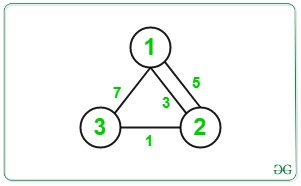Minimum Cost from source node 1 to destination node N is = 3/2 + 1 = 1 + 1 = 2.

Input:_ N = 3, M = 3, Edges = {{2, 3, 1}, {1, 3, 7}, {2, 1, 5}}_

Output:_ 2_

Explanation: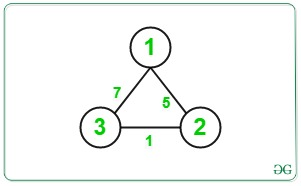Minimum Cost from source node 1 to destination node N is = 7/2 = 3.

## Recommended: Please try your approach on {IDE} first, before moving on to the solution.

Approach: The idea is to consider every edge and try to minimize the overall cost by reducing its cost. The main idea is to break the path between source to destination into the source to any vertex u i.e., path(1 to u) and from destination to any vertex v i.e., path(n to v) for all u and v. Below are the steps:

1. Perform a Dijkstra Algorithm to find the single source shortest path for all the vertex from source node 1 and store it in an array as dist_from_source[].
2. Perform a Dijkstra Algorithm to find the single source shortest path for all the vertex from source node N and store it in an array as dist_from_dest[].
3. Initialise the minimum cost(say minCost) as maximum value.
4. Traverse the given edges and for each edges reduce the current cost to half and update the minimum cost as:

minCost = min(minCost, dist_from_source[u] + c/2 + dist_from_dest[v])

where,

c is the cost of current edge,

dist_from_source[u] is cost of path from node 1 to u

dist_from_source[v] is cost of path from node v to N

#algorithms #graph #queue #dijkstra #priority-queue #algorithms